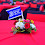## Saturday, November 17, 2018

### Financial Ratios: Much More Than Meets The Eye

Looking at financial ratios is part and parcel of the fundamental analysis process, where it is carried out to determine if a company’s share, REIT or corporate bond is worth to invest. For today’s post we shall look at the mathematical aspect of ratios and how we could gain more insights into these numbers.

Ratio Basics

While most financial ratios are given as numbers, it is the actual end result of a fraction. A ratio is just that, a fraction, and it is made up of a numerator and a denominator. A Price-to-Book (P/B) ratio of 0.8 could mean anything; it could be 0.80/1.00, or 1.20/1.50.

When comparing ratios across different time periods, it is important to look at the numerator and denominator, not just the ratios. Take for instance the dividend yield ratio of a fictitious Company A over three years below:

2015: 3.5%
2016: 4%
2017: 5%

At first glance it looks good, with an increasing dividend yield ratio over the three years. But if we look deeper at the numbers that results in the ratios, you may want to reconsider investing in Company A:

2015: (\$0.035/\$1.00) x 100% = 3.5%
2016: (\$0.032/\$0.80) x 100% = 4%
2017: (\$0.030/\$0.60) x 100% = 5%

In actual fact, Company A’s dividend amount and share price is reducing over the three years. So it is important that you should look at the make-up of the ratios instead of taking them at face value.

Another point on ratios is purely math 101; if a ratio is rising, it could mean one of the five possible reasons:

• The numerator is getting larger while the denominator remains the same.
• The numerator is getting larger while the denominator is getting smaller.
• The numerator is getting larger while the denominator is getting larger but at a slower pace than the former.
• The numerator remains the same while the denominator is getting smaller.
• The numerator is getting smaller while the denominator is getting smaller but at a faster pace than the former.

Thus if a ratio is falling, the five possible reasons are:

• The numerator is getting smaller while the denominator remains the same.
• The numerator is getting smaller while the denominator is getting larger.
• The numerator is getting smaller while the denominator is getting smaller but at a slower pace than the former.
• The numerator remains the same while the denominator is getting larger.
• The numerator is getting larger while the denominator is getting larger but at a faster pace than the former.

Fun With Financial Ratio Math

If you know your financial ratios well, you can probably see more things from just a simple ratio. For example, the Return on Equity (ROE) ratio of a company is simply:

ROE = Net Income / Average Shareholders’ Equity

Since shareholders’ equity is akin to book value (assets – liabilities), and net income is also known as (net) earnings, we will have this:

ROE = Earnings / Book Value

Here comes the interesting part.

If P/B = Price / Book Value, and Price-to-Earnings (P/E) = Price / Earnings, then:

ROE = Earnings / Book Value = (Price / Book Value) / (Price / Earnings) = (Price / Book Value) x (Earnings / Price)

Hence, ROE = (P/B) / (P/E)

In other words, we could also use the relationship between a company’s P/B and P/E ratios to analyse the ROE.

The Dupont Analysis

The Dupont analysis, which was named after the Dupont conglomerate (the makers of Teflon and Kevlar), is an in-depth look of the ROE ratio by splitting it into three main components; profit margin, total asset turnover and financial leverage.

Under the Dupont analysis, the ROE is:

ROE = Profit Margin x Total Asset Turnover x Financial Leverage = (Net Income / Sales) x (Sales / Assets) x (Assets / Equity)

Breaking it down further on the profit margin part, we have:

ROE = (Net Income / Pretax Income) x (Pretax Income / Earnings Before Interest and Taxes) x (Earnings Before Interest and Taxes / Sales) x (Sales / Assets) x (Assets / Equity)

By analyzing the ratios of these components, one can see which part is affecting or contributing to the resulting ROE.

Conclusion

I hope this post provides you with some general knowledge on financial ratios. Still, ratios are just one aspect in fundamental analysis; a complete and holistic picture is needed when researching and analysing your next company share, REIT or corporate bond.

#### 1 comment:

1.Your blog is too informative. Keep sharing your post in future too.I think people should acquire a lot from this weblog its really user pleasant. So much great information on here.
stock recommendation singapore